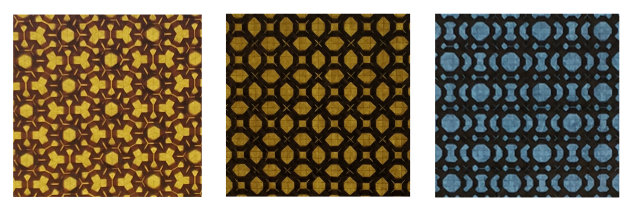# Groups in Galway 2021 — Abstracts

### Cristina Acciarri: A stronger version of Neumann's BFC-theorem

A celebrated theorem of B. H. Neumann states that if $G$ is a group in which all conjugacy classes are finite with bounded size, then the derived group $G'$ is finite. In this talk we will discuss a stronger version of Neumann's result and some consequences for finite groups and for profinite groups with restricted centralizers of $\pi$-elements. Based on a joint work with Pavel Shumyatsky.

### Jesús Hernández Hernández: Conjugacy classes of big mapping class groups

In this talk we will recall what are the big mapping class groups, and what differences are there between them and the usual mapping class groups. In particular, while in both cases we have a natural topology, the usual mapping class groups turn out to be discrete groups, while big mapping class groups become particularly interesting Polish groups. With this in mind, we will talk about our study of the topological behaviour of the conjugacy classes in big mapping class groups, from which we obtained the following results: all conjugacy classes are meager, and the characterisation of the existence of dense conjugacy classes. This is joint work with Michael Hrusak, Anja Randecker, Israel Morales, Manuel Sedano and Ferrán Valdez.

### Caroline Lassueur: On the trivial source character tables of finite groups

Trivial source modules play an important rôle in block theory, for example in the description of various equivalences between block algebras. A remarkable property of these modules lies in the fact that they lift from positive characteristic to characteristic zero and hence can be studied through ordinary characters. The trivial source character table (also called species table of the trivial source ring) contains a lot of information about these modules and their associated Brauer quotients. I will discuss recent results about these.

### Paula Lins: Twisted conjugacy for soluble matrix groups

In this talk we introduce twisted conjugacy and will see sufficient conditions for groups of soluble triangular matrices to have infinitely many twisted conjugacy classes. Our first approach reduces the investigation to a metabelian subgroup of $GL_2$, while our second tool shows how automorphisms of the base ring come into play when the solubility class is large enough. As corollary, we provide a series of examples of soluble arithmetic groups with property $R_\infty$, complementing or generalizing earlier results due to Taback–Wong, Gonçalves–Wong, Fel'shtyn–Gonçalves and Gonçalves–Kochloukova. This is a joint work with Yuri Santos Rego.

### Alastair Litterick: Geometric invariant theory and Generating Sets in Finite Simple Groups

It is by now a classical theorem that all non-abelian finite simple groups (FSGs) are $2$-generated. Various people have pushed this result in various directions, such as studying the probability of generating FSGs with random elements, or finding generators of particular orders, or from particular conjugacy classes, or satisfying particular relations.

When the FSGs in question are groups of Lie type, these questions can be expressed in terms of a representation variety $\mathrm{Hom}(F,G)$, where $F$ is finitely generated and $G$ is a simple algebraic group. In work with Ben Martin (Aberdeen), we interpret properties of $F$ and of the desired subgroups of $G$ in terms of algebraic geometry and geometric invariant theory. This lets us show, for instance, that if the generators of $F$ multiply to $1$ and a natural dimension condition holds in $G$, then only finitely many groups of Lie type $G(p^e)$ can be an image of $F$, for each prime $p$. Apart from its independent interest, this opens up a new avenue of attack in the wider study of generating sets in groups of Lie type.

### Rachel Quinlan: Pleats, twists and wallpaper: a backlit story

This talk reports on a recreational project at the intersection of group theory and mathematical art. The premise is that the art and craft of origami provide an excellent environment for exploring patterns with symmetry, mostly because of the extraordinary physical and mechanical properties of paper. We will discuss a way to realize all seventeen wallpaper groups through minor adjustments to two basic designs - one for the five examples with 3-fold rotations, and another for the rest.### Bernardo Rodrigues: $2$-modular representations of the Conway simple groups as binary codes

Parts of this talk are from joint work with Wolfgang Knapp, Mathematisches Institut, Universität Tübingen, Germany

In the talk we introduce and discuss an elementary tool from representation theory of finite groups for constructing linear codes invariant under a given permutation group $G$. The tool gives theoretical insight as well as a recipe for computations of generator matrices and weight distributions. In some interesting cases a classification of code vectors under the action of $G$ can be obtained. As explicit examples we examine binary codes related to the $2$-modular reduction of the Leech lattice and Conway groups.